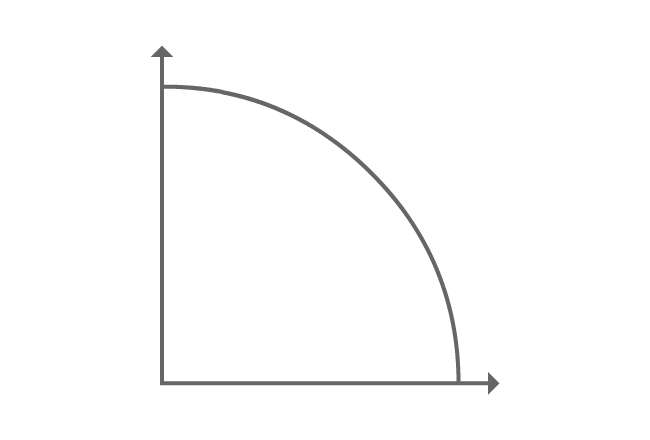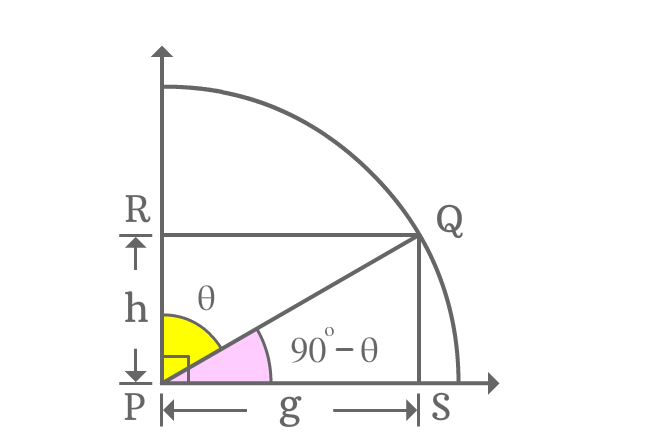# Cofunction identity of sin function

## Formula

Sexagesimal System
$\sin{(90^\circ-\theta)} \,=\, \cos{\theta}$
$\sin{\Big(\dfrac{\pi}{2}-x\Big)} \,=\, \cos{x}$

In this formula, the angle in degrees is denoted by Theta ($\theta$) and the angle in radians is denoted by $x$.

The angle of sin function is an allied angle in first quadrant. So, it is called as first quadrant’s allied angle identity of sin function. The angle of sin function is complement of the angle of the cos function. Therefore, it is also called as cofunction identity of sin function.

### ProofConsider first quadrant. A line segment is rotated to some angle and it is named as $\overline{PQ}$.

Draw perpendicular lines to both horizontal and vertical lines from $Q$ and they interest them at points $S$ and $R$ respectively. Thus, it forms two right angled triangles $\Delta QPS$ and $\Delta QPR$ in first quadrant.

Take $\angle QPR = \theta$, then $\angle QPS = 90^\circ-\theta$.

Take $PS = g$, then $RQ = g$. Similarly, take $RP = h$, then $QS = h$.

#### Express sin of allied angleThe angle of $\Delta QPS$ is an allied angle and it is $90^\circ-\theta$. Now, find the value of sine of first quadrant’s allied angle by expressing it in terms of ratio of the sides of the triangle.

$\sin{(90^\circ-\theta)} = \dfrac{SQ}{PQ}$

The length of the opposite side ($\overline{QS}$) is $h$ but the length of the hypotenuse is unknown. However, the length of the adjacent side is known. Therefore, the length of the hypotenuse can be calculated by Pythagorean theorem.

$\implies \sin{(90^\circ-\theta)} = \dfrac{h}{\sqrt{g^2+h^2}}$

#### Find equivalent value of the fractionIn previous step, sin of allied angle of first quadrant is expressed as a fraction. Now, calculate the value of the fraction in the form a trigonometric function.

It can be done by considering $\Delta QPR$.

$\dfrac{h}{\sqrt{g^2+h^2}} = \dfrac{RP}{PQ}$

As per $\Delta QPR$, the angle of the triangle is theta. The ratio of lengths of $\overline{RP}$ to $\overline{PQ}$ is cos of angle theta.

$\implies \dfrac{h}{\sqrt{g^2+h^2}} = \cos{\theta}$

#### Cofunction identity of sin function

Geometrically, the two steps have proved that

$\sin{(90^\circ-\theta)}$ $=$ $\dfrac{h}{\sqrt{g^2+h^2}}$ $=$ $\cos{\theta}$

$\therefore \,\,\,\,\,\, \sin{(90^\circ-\theta)} \,=\, \cos{\theta}$

Sin of allied angle of first quadrant is equal to the cos of angle. So, it is known first quadrant’s allied angle identity of sin function. The two angles of both trigonometric functions are complementary angles. So, the trigonometric identity is called as cofunction identity of sin function.

A best free mathematics education website that helps students, teachers and researchers.

###### Maths Topics

Learn each topic of the mathematics easily with understandable proofs and visual animation graphics.

###### Maths Problems

A math help place with list of solved problems with answers and worksheets on every concept for your practice.

Learn solutions

###### Subscribe us

You can get the latest updates from us by following to our official page of Math Doubts in one of your favourite social media sites.